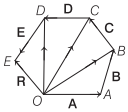# Addition of Vectors Definitions, formulas – Scalars and Vectors

## Addition of Vectors Definitions, Formulas – Scalars and Vectors

We are giving a detailed and clear sheet on all Physics Notes that are very useful to understand the Basic Physics Concepts.

1. Triangle Law of Vectors Addition:
If two vectors acting at a point are represented in magnitude and direction by the two sides of a triangle taken in one order, then their resultant is represented by the third side of the triangle taken in the opposite order.If two vectors A and B acting at a point are inclined at an angle 0, then their resultant

R = $$\sqrt{A^{2}+B^{2}+2 A B \cos \theta}$$

If the resultant vector R subtends an angle β with vector A, then

tan β = $$\frac{B \sin \theta}{A+B \cos \theta}$$

2. Parallelogram Law of Vectors Addition:
If two vectors acting at a point are represented in magnitude and direction by the two adjacent sides of a parallelogram draw from a point, then their resultant is represented in magnitude and direction by the diagonal of the parallelogram drawn from the same point.Resultant of vectors A and B is given by

R = $$\sqrt{A^{2}+B^{2}+2 A B \cos \theta}$$

If the resultant vector R subtends an angle β with vector A, then

tan β = $$\frac{B \sin \theta}{A+B \cos \theta}$$

3. Polygon Law of Vectors Addition:
It states that, if number of vectors acting on a particle at a time are represented in magnitude and direction by the various sides of an open polygon taken in same order, then their resultant vector is represented in magnitude and direction by the closing side of polygon taken in opposite order. In fact, polygon law of vectors is the outcome of triangle law of vectors.R = A + B + C + D + E
OE = OA + AB + BC + CD + DE